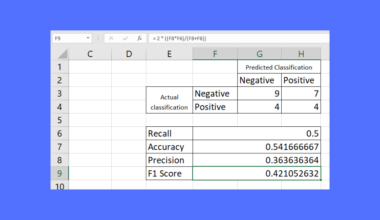# How to Calculate Hamming Distance in Excel

This guide will explain how to calculate the hamming distance in Excel using the `COUNT` function and the `SUMPRODUCT` function.

The rules for using the `SUMPRODUCT` function in Excel is the following:

• The `SUMPRODUCT` function will treat non-numeric values in selected arrays as zeros.
• The array arguments we input must be the same or equal in size. If not, the function will return a #VALUE! error value.
• When we include logical tests inside arrays, it will create TRUE and FALSE values. In this case, we want to coerce these logical values to 1’s and 0’s.
• The function often uses the result of other functions directly.

Since it has several built-in mathematical functions and features, Excel is a popular tool for statistical and mathematical calculations. With the help of built-in functions, we can easily perform difficult and complex calculations.

For instance, we can easily calculate the hamming distance in Excel. So hamming distance refers to a measure for contrasting two binary data strings. Essentially, we need to count the number of bits that differ between two values of the same length to get the hamming distance.

When we compare two binary strings of data that have identical lengths, the number of but locations where the two bits disagree or differ is the hamming distance. So we simply count the pair of adjacent numerals or locations that are different or distinct to determine the hamming distance.

And this may sound complicated. But, we can easily calculate this using a formula combining the `SUMPRODUCT` function and the `COUNT` function in Excel.

Let’s take a sample scenario wherein we need to calculate the hamming distance in Excel.

Suppose you have two vectors. And you want to determine the hamming distance between the two of them. So you used a formula wherein the `COUNT` function to find the total number of observations in the data set.

Then, the formula compares each pair observation between the columns and returns a logical value. Next, it will convert the TRUE or FALSE to 0 and 1. Lastly, the `SUMPRODUCT` function will find the sum of all the 1’s.

Before we move on to a real example, let’s first learn how to write the functions we will utilize.

## The Anatomy of the SUMPRODUCT Function

The syntax or the way we write the `SUMPRODUCT` function is as follows:

`=SUMPRODUCT(array1, [array2])`

Let’s take apart this formula and understand what each term means:

• = the equal sign is how we activate any function in Excel.
• SUMPRODUCT() refers to our `SUMPRODUCT` function. And this function is used to return the sum of the products of the corresponding ranges or selected arrays.
• array1 is a required argument. So it refers to 2 up to 255 arrays for which we want to multiply and add the components. Additionally, all the selected arrays must have the same or equal dimensions.
• array2 is an optional argument. And this is only a supplement to the arrays already selected in array1. So it still refers to 2 to 255 arrays we want to perform the function to.

## The Anatomy of the COUNT Function

The syntax or the way we write the `COUNT` function is as follows:

`=COUNT(value1, [value2])`

Let’s take apart this formula and understand what each term means:

• = the equal sign is how we begin any function in Excel.
• COUNT() is our `COUNT` function. And this function is used to count the number of cells in a range that contains numbers.
• value1 is a required argument. So this refers to 1 to 255 arguments that can contain or refer to different types of data. However, only numbers are counted.
• value2 is an optional argument. And this again refers to 1 to 255 arguments that have a variety of different types of data. But, the function only counts numbers.

Great! Now we can dive into a real example of calculating hamming distance in Excel.

## A Real Example of Calculating Hamming Distance in Excel

Let’s say we have a data set containing two binary data values. And we want to calculate the gaming distance between the binary values. So our initial data set would look like this: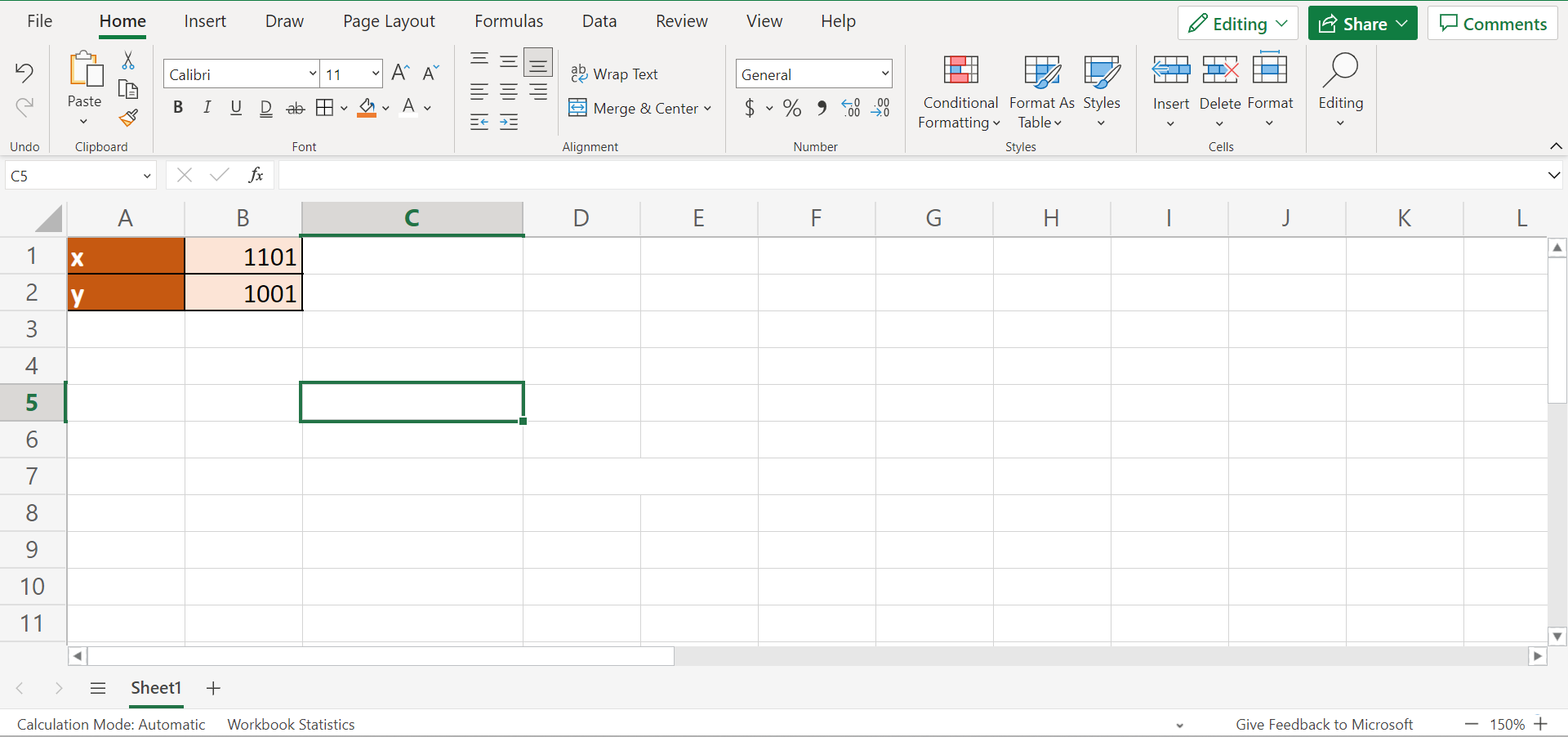For example, our two binary values are `x = 1101` and `y = 1001`. So we will input the values in columns to display them as vectors. Then, we can apply our formula by combining the `COUNT` and `SUMPRODUCT` functions to get the hamming distance between the two binary data.

So the `COUNT` function is used to determine the number of entries there are in the data set that is part of the range or array of numbers. Moreover, this will identify how many cells in the data set have a number in them.

And the `SUMPRODUCT` function will return the total of the sums of the products of the comparable ranges.

Firstly, the `COUNT` function will find the total number of observations in the selected column. Then, the formula will compare the two columns to check whether each pair matches. In this case, the output will be a logical value of TRUE or FALSE.

Next, the formula has hyphens which will convert the TRUE or FALSE into 1s or 0s. Afterward, the `SUMPRODUCT` function will calculate the total sums of the array. Lastly, the formula will subtract the results to return the hamming distance.

Additionally, we can also apply the same formula to calculate the hamming distance between two numeric vectors. For instance, we have `x = [3, 7, 8, 10]` and `y = [2, 6, 8, 10]`. Since the two vectors have different values, we would get a two-element difference in the hamming distance.

Similarly, we will input the values in columns to represent them as vectors. Then, we can apply the same formula to calculate the hamming distance between two numerical vectors.

And our final data set would look like this: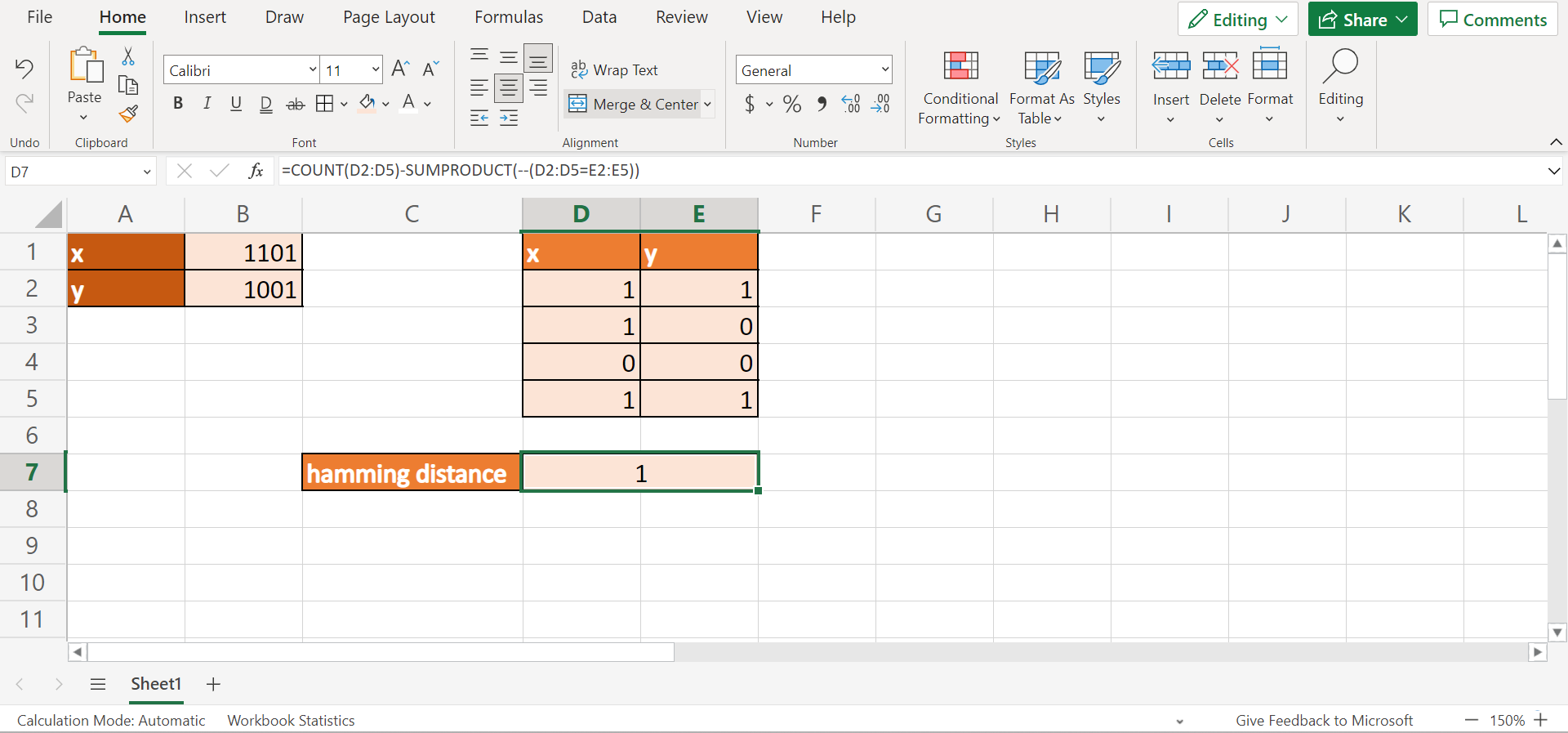Amazing! Now we can dive into the steps of how to calculate the hamming distance in Excel using the `COUNT` function and the `SUMPRODUCT` function.

## How to Calculate Hamming Distance in Excel

In this section, we will explain the step-by-step process of how to calculate the hamming distance in Excel. Additionally, each step contains detailed instructions and pictures to guide you through the process.

To apply this method, simply follow the steps below.

1. Firstly, we will input the values as columns to represent them as vectors. Then, we can start the formula by typing in an equal sign and writing “=COUNT(D2:D5)-SUMPRODUCT(–(D2:D5=E2:E5))”. Lastly, we will press the Enter key to return the result.2. And tada! We have successfully calculated the hamming distance in Excel using the `COUNT` and `SUMPRODUCT` functions.3. Secondly, let’s try getting the hamming distance between two numerical vectors. In this case, we will type them again as columns. Then, we will input the same formula “=COUNT(D10:D13)-SUMPRODUCT(–(D10:D13=E10:E13))”. After, we will press the Enter key to return the result.4. And tada! We have determined the hamming distance between two numerical vectors in Excel.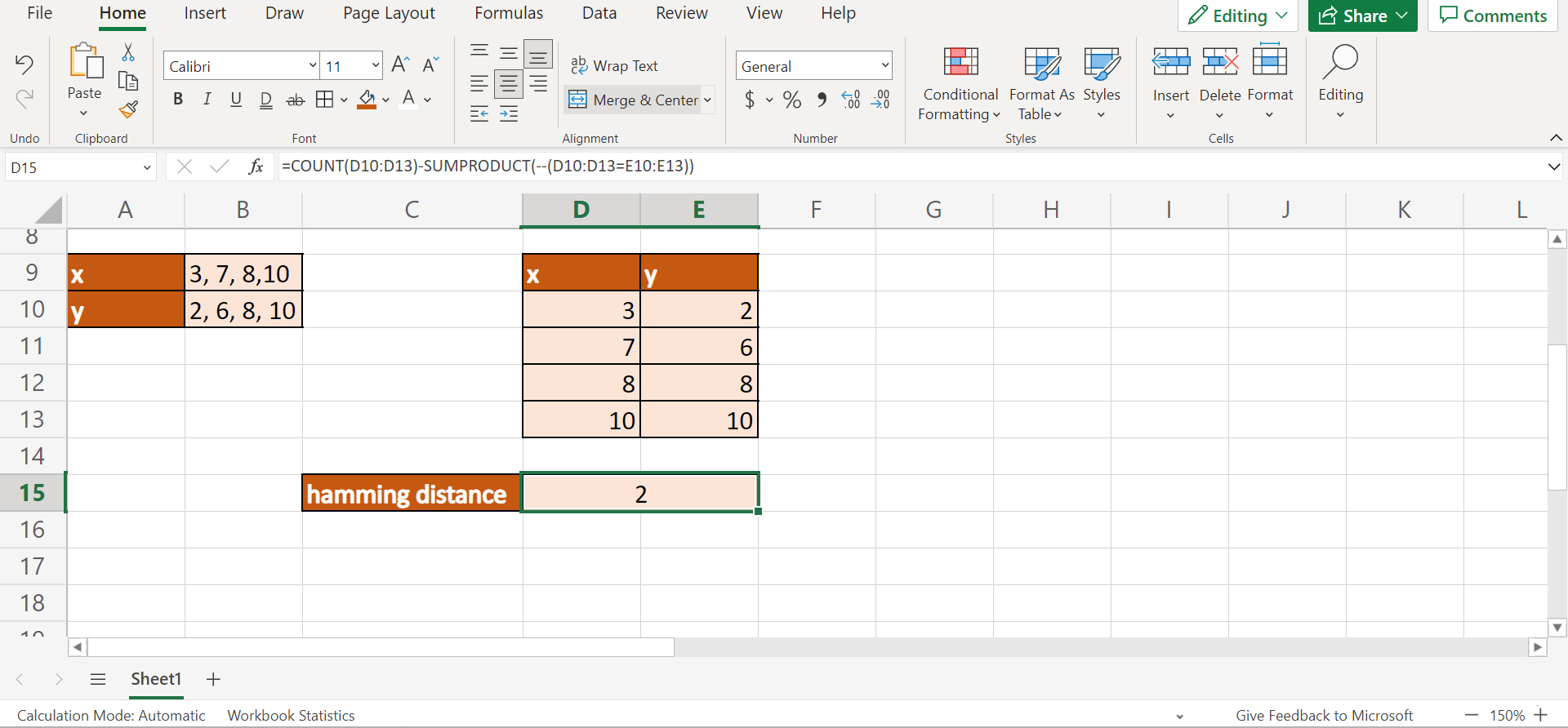And that’s pretty much it! We have successfully discussed how to calculate the hamming distance in Excel using the built-in functions. Now you can apply this method in your work whenever you need to get the hamming distance of two binary data or two numerical vectors.

Are you interested in learning more about what Excel can do? You can now use the `COUNT` function and the various other Microsoft Excel formulas available to create great worksheets that work for you. Make sure to subscribe to our newsletter to be the first to know about the latest guides and tutorials from us.Our goal this year is to create lots of rich, bite-sized tutorials for Google Sheets users like you. If you liked this one, you'll love what we are working on! Readers receive ✨ early access ✨ to new content.

##### You May Also Like## How to Create a Gauge Chart in Excel

The gauge chart in Excel is useful when you want to create an eye-catching visualization for a certain…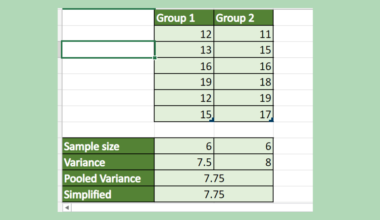## How to Calculate Pooled Variance in Excel

This guide will explain how to calculate pooled variance in Excel using the VAR.S function. The rules for…## How to Enable Macros in Excel

This guide will explain how to enable macros in your Excel spreadsheet. Excel macros are a powerful feature…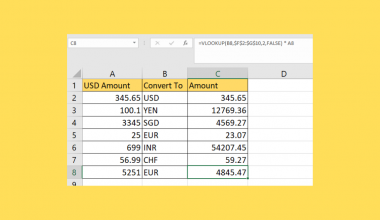## How to Do Currency Conversion using VLOOKUP in Excel

This guide will explain how we can perform currency conversion using the VLOOKUP function in Excel. The VLOOKUP…